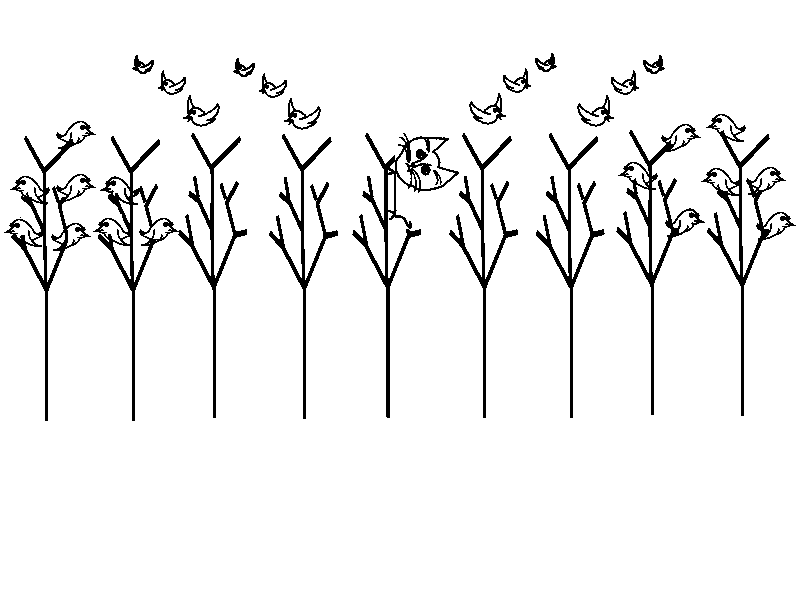MTH4300 Home

# MTH 4300: Final Practice 4

Problem 1.

What happens when the following program is executed? Provide a rigorous justification for your answer.

  #include<set>
#include<iostream>
int main(){
std::set<int> S;
S.insert(8);
S.insert(1);
S.insert(7);
S.insert(1);
S.insert(9);
S.insert(2);
S.insert(4);
S.insert(3);
S.insert(3);
S.insert(7);
std::cout<<S.size()<<std::endl;
return 0;
} 

Problem 2.

The user input consists of a positive integer $$n$$ followed by the sequence $$a=\left(a_0,a_1,\dots, a_{n-1}\right)$$ of $$n$$ positive integers. Calculate the largest of all the terms of the sequence $$a$$ that are divisible by $$22$$.

Example:

Input:
10
67 110 45 220 110 111 44 21 66 67
Output:
220


Problem 3.

What happens when the following program is executed? Provide a rigorous justification for your answer.

// myProg.cpp
// compile with
// c++ myProg.cpp -o myP -std=c++11
// execute with
// ./myP
#include<iostream>
int f(int& x){
x+=3;
return x;
}
int g(int* x){
x+=10;
return f(*x);
}
int main(){
int* x;
x=new int;
for(int i=0;i<100;++i){
x[i] = 8 * i + 3;
}
x=g(x);
std::cout<<x+x+x<<std::endl;
delete[] x;
return 0;
}


Problem 4.

What is printed on standard output when the following program is executed? Provide a rigorous justification for your answer.

 // mysteryPrinting.cpp
// compile with
// c++ mysteryPrinting.cpp -o mPrinting -std=c++11 -fno-elide-constructors
// execute with
// ./mPrinting
#include<iostream>
class Goblin{
private:
std::string name;
public:
Goblin(const std::string & = "");
Goblin(const Goblin &);
Goblin(Goblin &&);
void operator=(const Goblin &);
void operator=(Goblin &&);
virtual ~Goblin();
};
Goblin::Goblin(const std::string & s){
name=s;
}
Goblin::Goblin(const Goblin& x){
name="M"+x.name;
}
Goblin::Goblin(Goblin&& x){
name="N"+x.name;
x.name+="P";
}
void Goblin::operator=(const Goblin & x){
name+="Q"+x.name;
}
void Goblin::operator=(Goblin && x){
name+="R"+x.name;
x.name+="S";
}
Goblin::~Goblin(){
std::cout<<name<<std::endl;
}
Goblin sing(Goblin z){
Goblin w("T");
Goblin r=z;
return w;
}
int main(){
Goblin a("A");
Goblin b("B");
b=sing(a);
return 0;
} 

Problem 5.

Create the program TravelSummary that can produce the list of cities and countries that the user has visited. The user input consists of a positive integer $$n$$ followed by $$n$$ lines. Each line contains two strings: cityName and countryName. The program should list all countries in alphabetical order and for each of them print all different cities (also in alphabetical order). The format of the output is shown in the example below.

Example:
Input:
8
Ypres Belgium
Waterloo Belgium
Munich Germany
Antwerp Belgium
Frankfurt Germany
Output:
The user has been in Belgium and visited: Antwerp, Waterloo, Ypres
The user has been in Canada and visited: Ottawa, Waterloo
The user has been in Germany and visited: Frankfurt, Munich


Problem 6.

The user input consists of multiple lines. In each line the user provides a string mountain and a positive real number height that correspond to the names of mountains and their heights (in meters). A negative number in one of the lines means that the input is over. The last line should not be taken into account. Determine the longest subsequence of consecutive lines from the user input in which every mountain is taller than three thousand meters.

Example:
Input:
OlympusMons 21287.4
Kilauea 1247
MountEverest 8848
Kilimanjaro 5895
MontBlanc 4808.7
AppalachianMountains 2037
MountRainier 4392
Lassen 3187
LastLine -8

Output:
MountEverest 8848
Kilimanjaro 5895
MontBlanc 4808.7


Problem 7.

There are $$n$$ equally spaced trees in a row. They are labeled by $$0$$, $$1$$, $$\dots$$, $$n-1$$. For each $$i\in\{0,1,2, \dots, n-1\}$$, the tree $$i$$ contains $$b_i$$ birds. During the night a cat chooses one of the trees, climbs it, and eats all of the birds on that tree. However, in the morning all of the birds from the two closest trees on the left and all of the birds from the two closest trees on the right will fly away and never come back. For example the two closest trees to the left of the tree with label $$17$$ have labels $$15$$ and $$16$$. Similarly, the two closest trees to the right from the tree $$17$$ have labels $$18$$ and $$19$$. If the cat climbs and eats all of the birds on the tree $$17$$, then the trees $$15$$, $$16$$, $$17$$, $$18$$, and $$19$$ remain with $$0$$ birds each.The user input consists of the positive integer $$n$$ and the sequence $$b_0$$, $$b_1$$, $$\dots$$, $$b_{n-1}$$ of positive integers. Create the program of complexity $$O(n)$$ that outputs the maximal number of birds that the cat can eat.

Example:


Input:
10
25 1 1 2 25 1 30 25 1 2
Output:
75


Explanation: In this problem we have $$b_0=25$$, $$b_1=1$$, $$b_2=1$$, $$b_3=2$$, $$b_4=25$$, $$b_5=1$$, $$b_6=30$$, $$b_7=25$$, $$b_8=1$$, $$b_9=2$$. The first night the cat can eat the $$25$$ birds from the tree with label $$0$$. In addition to the tree $$0$$, the trees with labels $$1$$ and $$2$$ remain without birds. The second night the cat can eat the birds from the tree $$7$$ that has $$25$$ birds. In addition to the tree $$7$$, the trees $$5$$, $$6$$, $$8$$, and $$9$$ remain without birds. The third night the cat climbs the tree $$4$$ and eats all of the $$25$$ birds there. The total number of birds that the cat ate is $$75$$. With this configuration of trees the cat cannot eat more than $$25$$ birds.